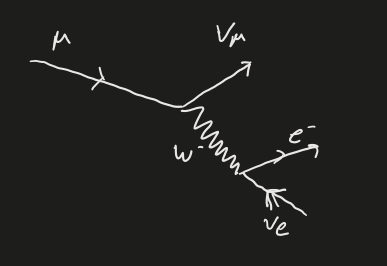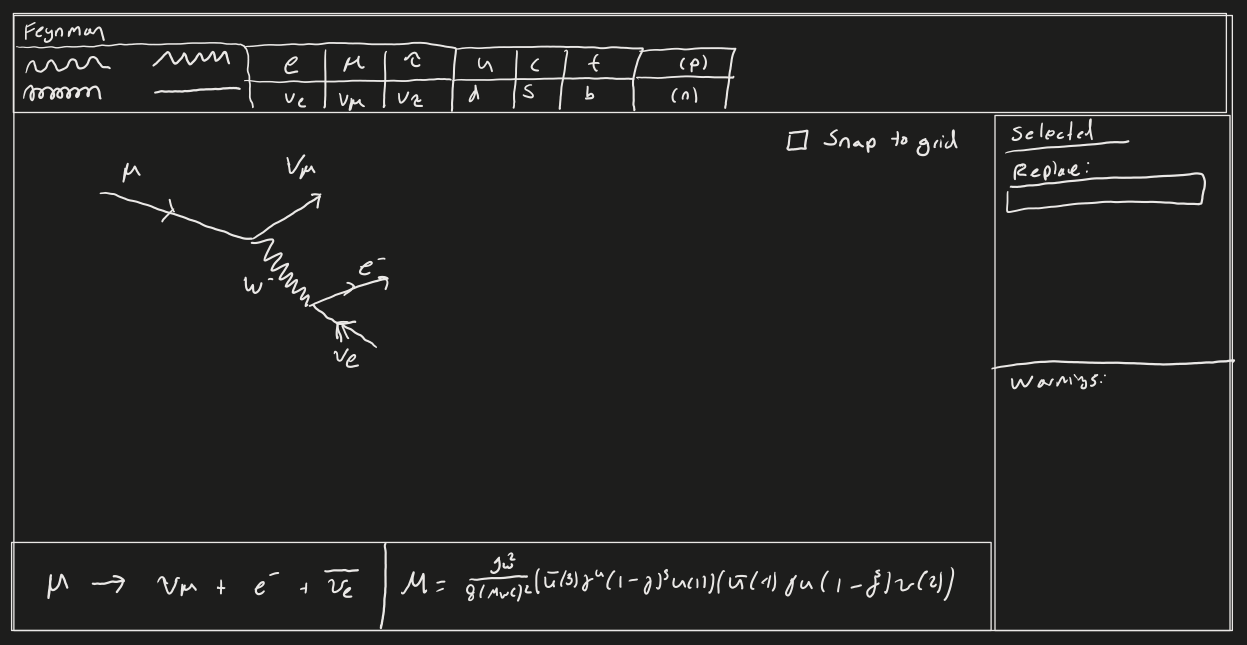# Feynman Diagram Editor Pt. 1

Starting a new project for validating Feynman diagrams.

## Overview

I’m currently reading “Introduction to Elementary Particles” by Griffiths. (Yes, the Griffiths, I love his books).

One of the projects I’ve wanted to do for awhile is build a Feynman diagram editor. But I want it to be more than just a tool for drawing squiggly lines, but something that could validate and provide insight on the digram.

The last two days were spent on laying some of the foundation for that application.

## Feynman Diagrams

Feynman diagrams are a nifty little tool for visualizing (and calculating) particle interactions.In a typical diagram, time flows from the left to the right, and one dimension of space is on the Y-axis (up and down). But, you may notice there’s no labels on the diagram. The diagram isn’t concerned with “how fast” or “how far” the interaction takes place. It’s actually a tool for counting (I’ll cover this in a future blog post).

Elementary particle collisions follow a number of rules. For example, charge must be conserved. So, if before a collision there’s +2 charge, after the collision there must be +2 charge.

Other things must be conserved such as “Baryon Number”, which is simply the number of Baryons (things like protons/neutrons, with 3 quarks). Or Lepton Generation Number (“electron-ness” must be conserved.)

So for stage one, I figured it’d be fun to build a program that validates different interactions.

## Tool

Right now it’s just a library right here:

But the library supports parsing interactions and then validating them for a few conservation laws. I still need to support spin, but I don’t quite understand spin conservation yet, so I need to re-read Ch 4.

let i1 = ParseInteraction("PROTON + ANTIPROTON -> PION_PLUS + PION")
expect(hasError(validateInteraction(i1), "CHARGE_CONSERVATION")).to.be.true;

let i2 = ParseInteraction("ETA_MESON -> PHOTON + PHOTON")
expect(hasError(validateInteraction(i2), "CHARGE_CONSERVATION")).to.be.false;

let i3 = ParseInteraction("SIGMA -> LAMBDA + PION")
expect(hasError(validateInteraction(i3), "ENERGY_CONSERVATION")).to.be.true;

let i4 = ParseInteraction("SIGMA_MINUS -> NEUTRON + PION_MINUS")
expect(hasError(validateInteraction(i4), "CHARGE_CONSERVATION")).to.be.false;
expect(hasError(validateInteraction(i4), "BARYON_CONSERVATION")).to.be.false;
expect(hasError(validateInteraction(i4), "ENERGY_CONSERVATION")).to.be.false;

let i5 = ParseInteraction("MUON -> ELECTRON + ELECTRON_ANTINEUTRINO")
expect(hasError(validateInteraction(i5), "LEPTON_MUON_CONSERVATION")).to.be.true;

let i6 = ParseInteraction("ELECTRON + PROTON -> ELECTRON_NEUTRINO + PION")
expect(hasError(validateInteraction(i6), "BARYON_CONSERVATION")).to.be.true;

let i7 = ParseInteraction("PION_PLUS + NEUTRON -> PION_MINUS + PROTON")
expect(hasError(validateInteraction(i7), "CHARGE_CONSERVATION")).to.be.true;

let i8 = ParseInteraction("SIGMA_PLUS + NEUTRON -> SIGMA_MINUS + PROTON")
expect(hasError(validateInteraction(i7), "CHARGE_CONSERVATION")).to.be.true;


## Diagram

Eventually I’m thinking the tool will look something like this:With a couple of features:

• Snap-to-grid, no messy diagrams
• All client-side with URL sharing
• Interaction names, bunch of examples
• Validation window, “charge not conserved”, etc
• Maybe calculating the interaction terms
• Maybe calculate different orders of interactions

## Future

Next up is the rest of the conservation laws, better vertex validation, and then I’ll start on the graphical editor!